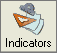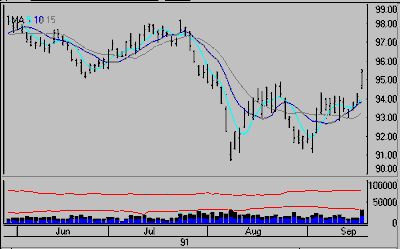Moving Average Previous  Top  Next

A moving average is a mathematical attempt to smooth fluctuations in price. It is the mean average of a price field (in this case, the close) over a given period. This is the sum of the prices over the period divided by the number of samples in the study. Each day the average is re-calculated using the same number of observations by adding one new reading and deleting the first reading for the period. Hence, the average is moving. This study is used to compare current values with one or more smoothed values. This is a moving average crossover study, which displays averages based on three intervals of your choice.To add moving averages to any price series, simply make a chart of the desired commodity contract, stock or index, click the "Indicator" button on the toolbar, and select "Moving Average."

Alternately, pull down the Chart menu, click "Study Toolbar" and select "Moving Average."

1st Period, 2nd Period and 3rd Period:

Prompts for moving average parameters will immediately be added to UA's "Select Study" screen. The user-defined parameters are the three moving average intervals, defined as the 1st Period, 2nd Period and 3rd Period, respectively. If fewer than three averages are desired, you must repeat a period value in the unused text box(es).

The default parameters are for 5-, 10- and 15-day moving averages, as used in the following example:Interpretation:

A rising moving average is associated with a rising market and a falling moving average is associated with a falling market. Since moving averages lag behind the market, some trend-followers buy when price rises above the moving average and sell when price falls below the moving average.

Other traders use multiple moving averages to generate trading signals from dual or triple-average crossovers. Buy signals occur when the shorter moving average crosses over the longer moving average. Sell signals require the reverse. Moving average crossover dynamics explain hindsight momentum changes in the market studied.